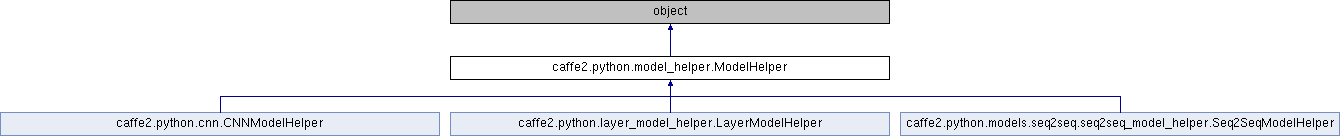Caffe2 - Python API A deep learning, cross platform ML framework
caffe2.python.model_helper.ModelHelper Class Reference
Inheritance diagram for caffe2.python.model_helper.ModelHelper:## Public Member Functions

def __init__ (self, name=None, init_params=True, allow_not_known_ops=True, skip_sparse_optim=False, param_model=None, arg_scope=None)

def arg_scope (self)

def get_name (self)

def create_param (self, param_name, shape, initializer, tags=None)

def get_param_info (self, param)

def add_param_DEPRECATED (self, param, key=None, shape=None, length=None)

def GetParams (self, namescope=None, top_scope=False)

def Proto (self)

def InitProto (self)

def RunAllOnGPU (self, args, kwargs)

def CreateDB (self, blob_out, db, db_type, kwargs)

def GetOptimizationParamInfo (self, params=None)

def Validate (self)

def GetComputedParams (self, namescope=None)

def GetAllParams (self, namescope=None)

def TensorProtosDBInput (self, unused_blob_in, blob_out, batch_size, db, db_type, kwargs)

def GetDevices (self)

def __getattr__ (self, op_type)

def __dir__ (self)

def GetCompleteNet (self)

def ConstructInitTrainNetfromNet (self, net)

## Public Attributes

name

net

param_init_net

params

init_params

allow_not_known_ops

skip_sparse_optim

weights

biases

## Detailed Description

```A helper model so we can manange models more easily. It contains net def
and parameter storages. You can add an Operator yourself, e.g.

model = model_helper.ModelHelper(name="train_net")
# init your weight and bias as w and b
w = model.param_init_net.XavierFill(...)
b = model.param_init_net.ConstantFill(...)
fc1 = model.FC([input, w, b], output, **kwargs)

or you can use helper functions in brew module without manually
defining parameter initializations and operators.

model = model_helper.ModelHelper(name="train_net")
fc1 = brew.fc(model, input, output, dim_in, dim_out, **kwargs)```

Definition at line 76 of file model_helper.py.

## Member Function Documentation

 def caffe2.python.model_helper.ModelHelper.__getattr__ ( self, op_type )
`Catch-all for all other operators, mostly those without params.`

Definition at line 425 of file model_helper.py.

 def caffe2.python.model_helper.ModelHelper.create_param ( self, param_name, shape, initializer, tags = `None` )
```Creates parameter with a given name and initializer.

If param_name is instance of BlobRefernce - then this blob will be used
to store parameter (no any logic will affect it's location).

If param_name is instance of a string type, then the final blob will
be created in the CurrentNameScope with the respect of all parameter
sharing logic, i.e. 'resolved_name_scope/param_name'.

Parameter sharing logic is going to override CurrentNameScope accoring
to the rules that are specified through ParameterSharing contexts,
all ParameterSharing contexts are applied recursively until there are no
extra overrides present, where on each step the best match will be
applied first.

The following examples should clarify the way ParameterSharing logic
works:

As an example if this function is called with parameter 'w':
a. Call from some scope 'global_scope' with no Parameter sharing:
'global_scope/w'
b. Call from scope 'scope_b', with override {'scope_b': 'scope_a'}:
'scope_a/w'
c. Call from scope 'scope_a', with override {'scope_a': ''}:
'scope_a/w'
d. Call from scope 'scope_b/shared', with overrides
{'scope_b/shared': 'scope_b', 'scope_b': 'scope_a'}:
'scope_a/w'
d. Call from scope 'scope_b/unshared', with overrides
{'scope_b/shared': 'scope_b', 'scope_b': 'scope_a'}:
'scope_a/unshared/w'
```

Definition at line 162 of file model_helper.py.

 def caffe2.python.model_helper.ModelHelper.get_param_to_grad ( self, params )
```Given a list of parameters returns a dict from a parameter
```

Definition at line 334 of file model_helper.py.

 def caffe2.python.model_helper.ModelHelper.GetComputedParams ( self, namescope = `None` )
```Returns the computed params in current namescope. 'Computed params'
are such parameters that are not optimized via gradient descent but are
directly computed from data, such as the running mean and variance
of Spatial Batch Normalization.
```

Definition at line 391 of file model_helper.py.

 def caffe2.python.model_helper.ModelHelper.GetOptimizationParamInfo ( self, params = `None` )
```Returns a map for param => grad.
If params is not specified, all parameters will be considered.
```

Definition at line 350 of file model_helper.py.

 def caffe2.python.model_helper.ModelHelper.GetParams ( self, namescope = `None`, top_scope = `False` )
```Returns the params in current namescope
```

Definition at line 283 of file model_helper.py.

 def caffe2.python.model_helper.ModelHelper.TensorProtosDBInput ( self, unused_blob_in, blob_out, batch_size, db, db_type, kwargs )
`TensorProtosDBInput.`

Definition at line 411 of file model_helper.py.

The documentation for this class was generated from the following file: